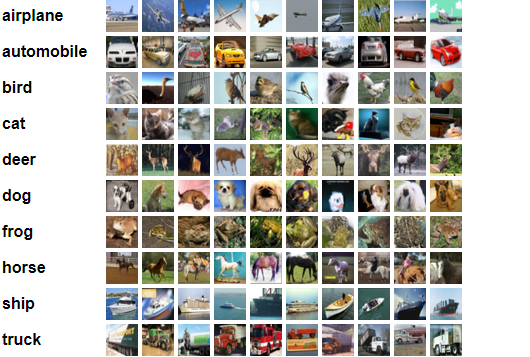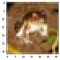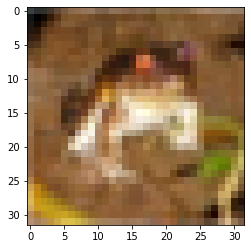1596990660

Image classification with PyTorch

CIFAR 10 Data set using logistic regressionIn my previous posts we have gone through

Let us try to solve image classification of CIFAR-10 data set with Logistic regression.

Step 1 : Import necessary libraries & Explore the data set

We are importing the necessary libraries pandas , numpy , matplotlib ,torch ,torchvision. With basic EDA we could infer that CIFAR-10 data set contains 10 classes of image, with training data set size of 50000 images , test data set size of 10000.Each image is of [3 x 32 x 32 ]. Which represents 3 channels RGB,32 x 32 pixel size.

#Explore CIFAR Data set
test_dataset = CIFAR10(root='data/', train=False, transform=ToTensor())
#size of training data
dataset_size = len(dataset)
dataset_size
#size of test data
test_dataset_size = len(test_dataset)
test_dataset_size
#number of classes in the data set
classes = dataset.classes
classes

Visualizing a sample image and the size of the sample image.

#Let us understand the size of one image
img, label = dataset
img_shape = img.shape
img_shape
#Let us look at a sample image
img, label = dataset
plt.imshow(img.permute((1, 2, 0)))
print('Label (numeric):', label)
print('Label (textual):', classes[label])

As this is a 3 channel RGB image Pytorch expects the channels as first dimension where as matplotlib expects as last dimension of the image.Here .permute tesnor method is used to shift channels to last dimesnionLabel (numeric): 6

Label (textual): frog

Step 2 : Prepare data for training

We using training set , validation set , Test set. Why we need them ?

Training set : used to train our model,computing loss & adjust weights Validation set : To evaluate the model with hyper parameters & pick the best model during training. we are using 10% of training data as validation set Test data set : Used to compare different models & report the final accuracy.

We are using the random_split from pytorch for creating train_ds,val_ds. torch.manual_seed(43) is set for reproducing the results.

#validation set size 5000 ie 10%
torch.manual_seed(43)
val_size = 5000
train_size = len(dataset) - val_size
#creating training & validation set using random_split
train_ds, val_ds = random_split(dataset, [train_size, val_size])
len(train_ds), len(val_ds)

We are using the data loader as we used in our previous example , with a batch size of 128. To visualize our data we are using make_grid helper function from torch vision.

#pytorch #neural-networks #image-classification #deep-learning #logistic-regression #deep learning

Buddha Community1591903440

Preprocessing your images for machine learning (image recognition)

During my studies at JKU there was a task for preprocessing images for a machine learning project. It is necessary to clean the raw images before using them in a learning algorithm, so thats why we create a pre-processing function. I think it can be quite useful for others as well so I want to share a bit of my approach. The file is structured in a way that it is easy to understand and also should have a tutorial-like effect.

#image-recognition #image #image-classification #machine-learning #image-processing1596990660

Image classification with PyTorch

CIFAR 10 Data set using logistic regressionIn my previous posts we have gone through

Let us try to solve image classification of CIFAR-10 data set with Logistic regression.

Step 1 : Import necessary libraries & Explore the data set

We are importing the necessary libraries pandas , numpy , matplotlib ,torch ,torchvision. With basic EDA we could infer that CIFAR-10 data set contains 10 classes of image, with training data set size of 50000 images , test data set size of 10000.Each image is of [3 x 32 x 32 ]. Which represents 3 channels RGB,32 x 32 pixel size.

#Explore CIFAR Data set
test_dataset = CIFAR10(root='data/', train=False, transform=ToTensor())
#size of training data
dataset_size = len(dataset)
dataset_size
#size of test data
test_dataset_size = len(test_dataset)
test_dataset_size
#number of classes in the data set
classes = dataset.classes
classes

Visualizing a sample image and the size of the sample image.

#Let us understand the size of one image
img, label = dataset
img_shape = img.shape
img_shape
#Let us look at a sample image
img, label = dataset
plt.imshow(img.permute((1, 2, 0)))
print('Label (numeric):', label)
print('Label (textual):', classes[label])

As this is a 3 channel RGB image Pytorch expects the channels as first dimension where as matplotlib expects as last dimension of the image.Here .permute tesnor method is used to shift channels to last dimesnionLabel (numeric): 6

Label (textual): frog

Step 2 : Prepare data for training

We using training set , validation set , Test set. Why we need them ?

Training set : used to train our model,computing loss & adjust weights Validation set : To evaluate the model with hyper parameters & pick the best model during training. we are using 10% of training data as validation set Test data set : Used to compare different models & report the final accuracy.

We are using the random_split from pytorch for creating train_ds,val_ds. torch.manual_seed(43) is set for reproducing the results.

#validation set size 5000 ie 10%
torch.manual_seed(43)
val_size = 5000
train_size = len(dataset) - val_size
#creating training & validation set using random_split
train_ds, val_ds = random_split(dataset, [train_size, val_size])
len(train_ds), len(val_ds)

We are using the data loader as we used in our previous example , with a batch size of 128. To visualize our data we are using make_grid helper function from torch vision.

#pytorch #neural-networks #image-classification #deep-learning #logistic-regression #deep learning1594537920

How to  Image Classification using PyTorch

In this post we will use a standard computer vision dataset - Dogs vs. Cats dataset that involves classifying photos as either containing a dog or cat.

Although, the dataset seems to be pretty simple, the goal would be to outline the steps required to solve image processing and classification using pytorch and the same pipeline can be later used to apply to any image classification problem at hand.

DataSet

The dogs vs cats dataset refers to a dataset used for a Kaggle machine learning competition held in 2013.

The dataset is comprised of photos of dogs and cats provided as a subset of photos from a much larger dataset of 3 million manually annotated photos. The dataset was developed as a partnership between Petfinder.com and Microsoft.

The dataset was originally used as a CAPTCHA (or Completely Automated Public Turing test to tell Computers and Humans Apart), that is, a task that it is believed a human finds trivial, but cannot be solved by a machine, used on websites to distinguish between human users and bots.

Let’s first call all the heavenly gods of python (import necessary libraries).

#deep-learning #image-classification #pytorch

1597565398

Laravel 7/6 Image Validation

In this image validation in laravel 7/6, i will share with you how validate image and image file mime type like like jpeg, png, bmp, gif, svg, or webp before uploading image into database and server folder in laravel app.

https://www.tutsmake.com/image-validation-in-laravel/

#laravel image validation #image validation in laravel 7 #laravel image size validation #laravel image upload #laravel image validation max #laravel 6 image validation1620200340

how to integrate CKEditor in Django

Welcome to my Blog, in this article we learn about how to integrate CKEditor in Django and inside this, we enable the image upload button to add an image in the blog from local. When I add a CKEditor first time in my project then it was very difficult for me but now I can easily implement it in my project so you can learn and implement CKEditor in your project easily.